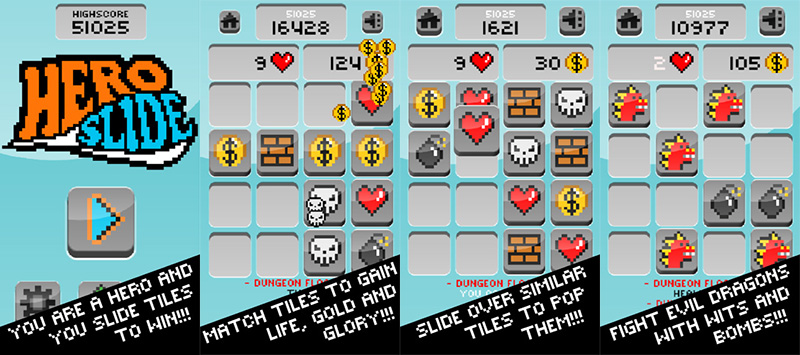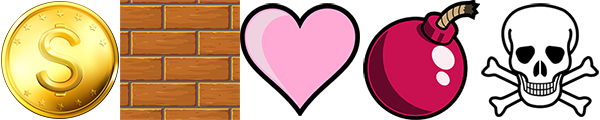# HTML5 prototype of iOS hit “Hero Slide” made with Phaser

Did you play Hero Slide? Awarded as TouchArcade Game of the Week, it’s a simple but clever game, half 2048 (read the tutorial series of this game) and half Dungeon Raid (read the tutorial series of this game too).It’s the right kind of game to create a tutorial series, and here it is with the first part, where we will learn how to: * Initialize the game * Place random tiles on the map * Move tiles with WASD keys I created some of the tiles in a single sprite sheet like this:Then you can play the first prototype with WASD key – focus the canvas first.
There is still some work to do as tiles do not match, but the main engine which controls tile movement is ok. Have a look at the fully commented source code:
```// the game
var game;

// size of each tile, in pixels
var tileSize = 120;

// different kinds of tiles
var tileTypes = 5;

// the game array, the board will be stored here
var gameArray = [];

// field size, in tiles. This will represent a 4x4 tiles field
var fieldSize = 4;

// creation of the game
game = new Phaser.Game(480, 480);
game.state.start("PlayGame");
}

var playGame = function(game){}

playGame.prototype = {

},
create: function(){

// initializing game board
for(var i = 0; i < fieldSize; i++){
gameArray[i] = [];
for(var j = 0; j < fieldSize; j++){

// each array item is an object with a tile value (0: empty) and a sprite (null: no sprite)
gameArray[i][j] = {
tileValue : 0,
tileSprite: null
};
}
}

// function to add a new item, will be explained later

// liteners to handle WASD keys. Each key calls handleKey function
},

// this function will add a new item to the board

// emptySpots is an array which will contain all the available empty tiles where to place a new item
var emptySpots = [];

// now we loop through the game board to check for empty tiles
for(var i = 0; i < fieldSize; i++){
for(var j = 0; j < fieldSize; j++){

// remember we define an empty tile as a tile whose tileValue is zero
if(gameArray[i][j].tileValue == 0){

// at this time we push a Point with tile coordinates into emptySpots array
emptySpots.push(new Phaser.Point(j, i));
}
}
}

// newSpot is a randomly picked item in emptySpots array
var newSpot = Phaser.ArrayUtils.getRandomItem(emptySpots);

// if newSpot is not null this means we have a place where to put a new tile
if(newSpot != null){

// selecting a random value between 1 and tileTypes
var tileType = game.rnd.between(1, tileTypes);

// updating game array with the new tile value and sprite
gameArray[newSpot.y][newSpot.x] = {
tileValue: tileType,
tileSprite: game.add.sprite(newSpot.x * tileSize, newSpot.y * tileSize, "tiles", tileType - 1)
}

// now the player can move
this.canMove = true;
}
},

// this function handles player movements
handleKey: function(e){

// first of all, let's see if the player can move
if(this.canMove){

// if the player can move, let's set canMove to false to prevent the player to move twice
this.canMove=false;

// time to check for the keycode which generated the event
switch(e.keyCode){

// "A" key (left)
case Phaser.Keyboard.A:

// we scan for game field, from TOP to BOTTOM and from LEFT to RIGHT starting from the 2nd column
for(var i = 0; i < fieldSize; i++){
for(var j = 1; j < fieldSize; j++){

// we can move a tile if it's not empty and the tile on its left is empty
if(gameArray[i][j].tileValue != 0 &amp;&amp; gameArray[i][j - 1].tileValue == 0){

// moving the sprite on the left
gameArray[i][j].tileSprite.x -= tileSize;

// copying the content of the current tile on the tile on its left
gameArray[i][j - 1] = {
tileValue: gameArray[i][j].tileValue,
tileSprite: gameArray[i][j].tileSprite
}

//setting current tile to empty
gameArray[i][j] = {
tileValue: 0,
tileSprite: null
}
}
}
}
break;

// "W" key (up)
case Phaser.Keyboard.W:

// we scan for game field, from TOP to BOTTOM and from LEFT to RIGHT starting from the 2nd row
// applying the same concepts seen before
for(var i = 1; i < fieldSize; i++){
for(var j = 0; j < fieldSize; j++){
if(gameArray[i][j].tileValue != 0 &amp;&amp; gameArray[i - 1][j].tileValue == 0){
gameArray[i][j].tileSprite.y -= tileSize;
gameArray[i - 1][j ] = {
tileValue: gameArray[i][j].tileValue,
tileSprite: gameArray[i][j].tileSprite
}
gameArray[i][j] = {
tileValue: 0,
tileSprite: null
}
}
}
}
break;

// "D" key (right)
case Phaser.Keyboard.D:

// we scan for game field, from TOP to BOTTOM and from RIGHT to LEFT starting from the next-to-last column
// applying the same concepts seen before
for(var i = 0; i < fieldSize; i++){
for(var j = fieldSize - 2; j &gt;= 0; j--){
if(gameArray[i][j].tileValue != 0 &amp;&amp; gameArray[i][j + 1].tileValue == 0){
gameArray[i][j].tileSprite.x += tileSize;
gameArray[i][j + 1] = {
tileValue: gameArray[i][j].tileValue,
tileSprite: gameArray[i][j].tileSprite
}
gameArray[i][j] = {
tileValue: 0,
tileSprite: null
}
}
}
}
break;

// "S" key (down)
case Phaser.Keyboard.S:

// we scan for game field, from BOTTOM to TOP and from LEFT to RIGHT starting from the next-to-last row
// applying the same concepts seen before
for(var i = fieldSize - 2; i &gt;= 0; i--){
for(var j = 0; j < fieldSize; j++){
if(gameArray[i][j].tileValue != 0 &amp;&amp; gameArray[i + 1][j].tileValue == 0){
gameArray[i][j].tileSprite.y += tileSize;
gameArray[i + 1][j ] = {
tileValue: gameArray[i][j].tileValue,
tileSprite: gameArray[i][j].tileSprite
}
gameArray[i][j] = {
tileValue: 0,
tileSprite: null
}
}
}
}
break;
}

// at the end of player input, we add a new item
}
}
}
```
Next time I am going to add animation to tile movement and the capability of matching tiles. Meanwhile download the source code.
214 GAME PROTOTYPES EXPLAINED WITH SOURCE CODE
// 1+2=3
// 10000000
// 2 Cars
// 2048
// Avoider
// Ballz
// Block it
// Blockage
// Bloons
// Boids
// Bombuzal
// Breakout
// Bricks
// Columns
// CubesOut
// Dots
// DROP'd
// Dudeski
// Eskiv
// Filler
// Fling
// Globe
// HookPod
// Hundreds
// InkTd
// Iromeku
// Lumines
// Magick
// MagOrMin
// Maze
// Memdot
// Nano War
// Nodes
// o:anquan
// Ononmin
// Pacco
// Phyballs
// Platform
// Poker
// Pool
// Poux
// Pudi
// qomp
// Racing
// Renju
// SameGame
// Security
// Sling
// Slingy
// Sokoban
// Splitter
// Sproing
// Stack
// Stringy
// Sudoku
// Tetris
// Threes
// Toony
// Turn
// TwinSpin
// vvvvvv
// Wordle
// Worms
// Yanga
// Zhed
// zNumbers